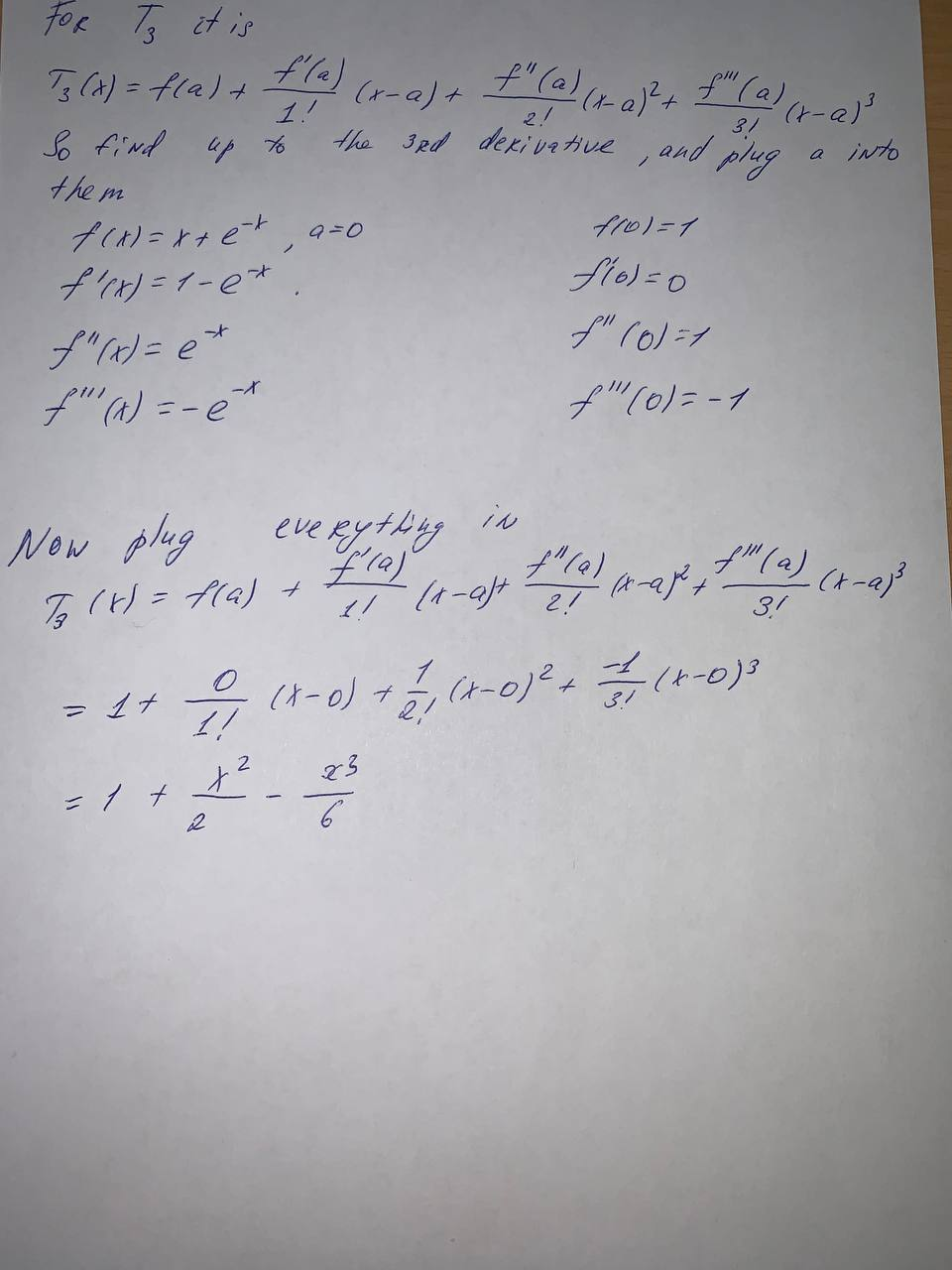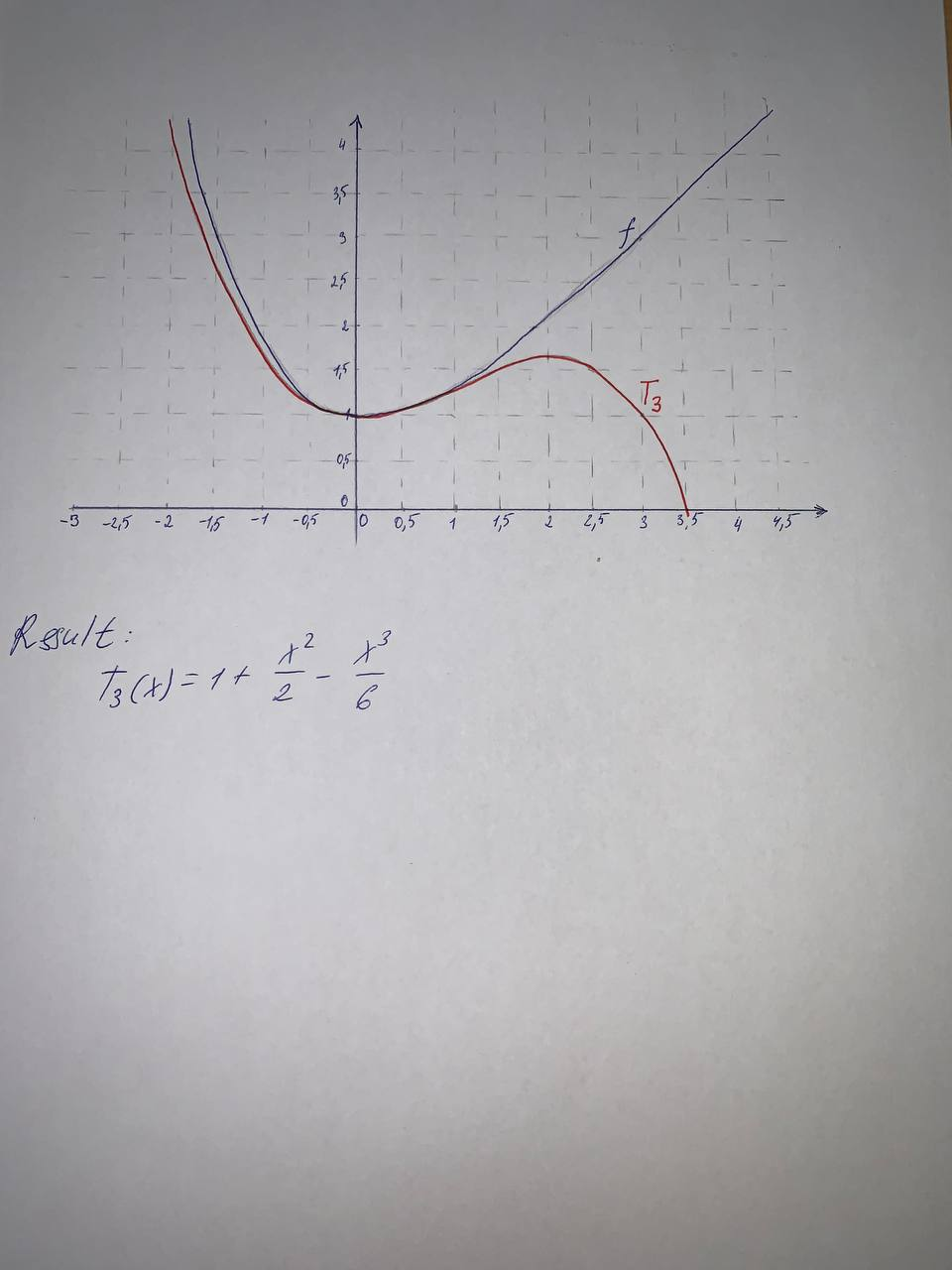Question# Find the Taylor polynomial T3(x) for the function f centered at the number a. Graph f and T3 on the same screen. f(x)=x+e^{-x}, a=0

Polynomial graphs
ANSWEREDFind the Taylor polynomial T3(x) for the function f centered at the number a. Graph f and T3 on the same screen.
$$f(x)=x+e^{-x}, a=0$$